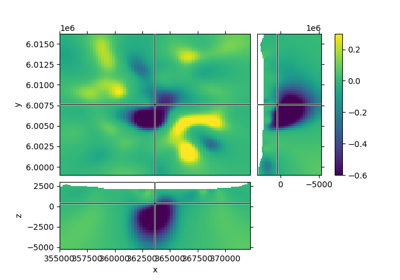# discretize.mixins.Slicer#class discretize.mixins.Slicer(mesh, v, xslice=None, yslice=None, zslice=None, v_type='CC', view='real', axis='xy', transparent=None, clim=None, xlim=None, ylim=None, zlim=None, aspect='auto', grid=[2, 2, 1], pcolor_opts=None, **kwargs)[source]#

Plot slices of a 3D volume, interactively (scroll wheel).

If called from a notebook, make sure to set

%matplotlib notebook

Parameters
v(`n_cells`) `numpy.ndarray`

Data array

xslice, yslice, zslice`float`, `optional`

Initial slice locations (in meter); defaults to the middle of the volume.

v_type: {‘CC’, ‘Fx’, ‘Fy’, ‘Fz’, ‘Ex’, ‘Ey’, ‘Ez’}

Type of visualization.

view{‘real’, ‘imag’, ‘abs’}

Which component to show.

axis{‘xy’, ‘yx’}

‘xy’: horizontal axis is x, vertical axis is y. Reversed otherwise.

transparent‘slider’ or `list` `of` `float` or `pairs` `of` `float`, `optional`

Values to be removed. E.g. air, water. If single value, only exact matches are removed. Pairs are treated as ranges. E.g. [0.3, [1, 4], [-np.infty, -10]] removes all values equal to 0.3, all values between 1 and 4, and all values smaller than -10. If ‘slider’ is provided it will plot an interactive slider to choose the shown range.

clim`None` or `list` `of` [`min`, `max`]

For pcolormesh (vmin, vmax). Note: if you use a norm (e.g., LogNorm) then vmin/vmax have to be provided in the norm.

xlim, ylim, zlim`None` or `list` `of` [`min`, `max`]

Axis limits.

aspect‘auto’, ‘equal’, `or` `num`

Aspect ratio of subplots. Defaults to ‘auto’.

A list of two values can be provided. The first will be for the XY-plot, the second for the XZ- and YZ-plots, e.g. [‘equal’, 2] to have the vertical dimension exaggerated by a factor of 2.

WARNING: For anything else than ‘auto’, unexpected things might happen

when zooming, and the subplot-arrangement won’t look pretty.

grid(3) `list` `of` `int`

Number of cells occupied by x, y, and z dimension on plt.subplot2grid.

pcolor_opts`dictionary`

Passed to pcolormesh.

Examples

The straight forward usage for the Slicer is through, e.g., a TensorMesh-mesh, by accessing its mesh.plot_3d_slicer.

If you, however, call this class directly, you have first to initiate a figure, and afterwards connect it:

```>>> fig = plt.figure()
```

Then you have to get the tracker from the Slicer

```>>> tracker = discretize.View.Slicer(mesh, Lpout)
```

Finally you have to connect the tracker to the figure

```>>> fig.canvas.mpl_connect('scroll_event', tracker.onscroll)
>>> plt.show()
```

Methods

 `onscroll`(event) Update index and data when scrolling. Update plot for change in Z-index. Update plot for change in Y-index. Update plot for change in X-index.

## Galleries and Tutorials using `discretize.mixins.Slicer`#Slicer demo

Slicer demo